# Gaussian Bayesian Network

## Introduction

A Bayesian network (BN) describes the joint probabilistic distribution of random variables through the conditional dependencies/independencies and presents as a graphical model (directed acyclic graph)(Scutari and Denis 2014). This provides an approach to decipher the probabilistic dependencies among variables of interest and has been applied in plant and animal breeding (Morota et al. 2012; Yu et al. 2019).

Recently, we published one paper about leveraging the Bayesian network (BN) to understand the interdependencies between rice phenotypes. I figured out that an example would be helpful for people who are interested in BN. In the following examples, I used bnlearn package (Scutari 2010) to infer the BN of 7 rice phenotypes. The example rice dataset is from Rice Diversity Panel 1, which measures Na+, K+, and Na+: K+ content in the roots and shoots (Zhao et al. 2011). Since all of these 7 phenotypes follow the Gaussian distribution, I specifically fitted a Gaussian Bayesian Network (GBN) here. In addition, I presented two different approaches to infer GBN: data-driven approach and the combination of the expert prior knowledge with data. The example rice dataset is available here.

library(bnlearn)
library(cowplot)
library(ggplot2)
library(gaston)
library(Rgraphviz)
library(MTM)

## Data import

# Import phenotype
pheno <- read.csv("salt_phenotypes.csv", row.names = 1)
dim(pheno) # 385   7
##  385   7
pheno <- na.omit(pheno) # remove NAs
dim(pheno) # 366   7
##  366   7
# Import genotype
geno <- read.bed.matrix("sativas413")
## Reading sativas413.fam
## ped stats and snps stats have been set.
## 'p' has been set.
## 'mu' and 'sigma' have been set.
dim(geno) # 413 36901
##    413 36901
## Subset accesions with both genotype and phenotype records.
geno@ped$id <- paste0("NSFTV_", geno@ped$id)
geno <- geno[geno@ped$id %in% row.names(pheno), ] dim(geno) # 363 36901 ##  363 36901 pheno <- pheno[match(geno@ped$id, row.names(pheno)), ]
dim(pheno) # 363   7
##  363   7
## Check if the ids of genotype and phenotype are identical
table(geno@ped$id == rownames(pheno)) ## ## TRUE ## 363 ## Construct genomic relationship matrix (GRM) # Quality control of genotype file with MAF & Callrate geno <- select.snps(geno, c(maf > 0.05 & callrate > 0.9)) dim(geno) # 363 29499 ##  363 29499 # Construct GRM matrix grm <- GRM(geno) dim(grm) # 363 363 ##  363 363 ## Fit multivariate mixed model to get estimated Breeding Values (EBV) # Define a MTM_func using MTM Package MTM_func <- function( Y, G, nTrait, nIter, burnIn, thin, prefix) { library(MTM) set.seed(007) # reproducible results MTM ( Y = Y, K = list ( list ( K = G, COV = list ( type = 'UN', df0 = nTrait, S0 = diag (nTrait) ) ) ), resCov = list ( type = 'UN', S0 = diag (nTrait), df0 = nTrait), nIter = nIter, burnIn = burnIn, thin = thin, saveAt = prefix) } Y <- scale(pheno, center = TRUE, scale = TRUE) G <- grm nTrait <- ncol(Y) MTM_fit <- MTM_func( Y = Y, G = G, nTrait = nTrait, nIter = 1200, burnIn = 200, thin = 5, prefix = 'MTM_fit') ## Check gibbs samples list.files(pattern = 'MTM_fit') ## Retrieve estimates str(MTM_fit) BV <- MTM_fit$K[]$U # estimated breeding values dim(BV) # 363 7 colnames(BV) <- colnames(pheno) BV <- as.data.frame(BV) ## Structure learning using EBV #------------------------- # Predefined function #------------------------- ## Check strength and direction in BN check_boot <- function(boot, Strength_Thres, Direction_Thres) { boot[(boot$strength >= Strength_Thres) & (boot\$direction > Direction_Thres),]
}

### Structure learning: data-driven approach

# Score-based algorithm: Tabu Search
tabu_simple <- tabu(BV)
graphviz.plot(tabu_simple, main = "Tabu_data-driven", shape = "ellipse",
layout = "dot")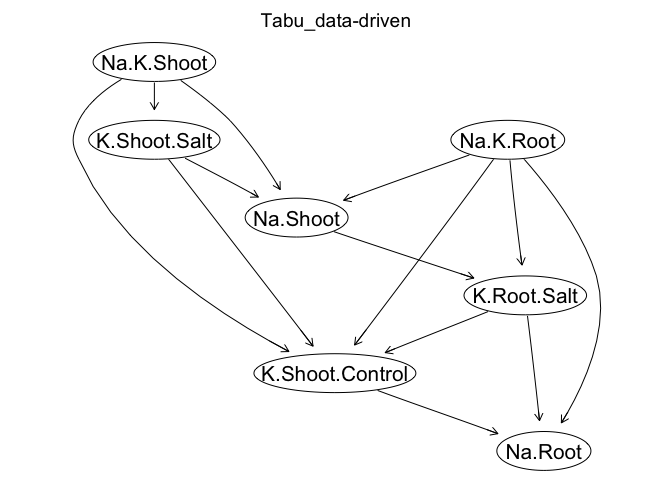# Model Averaging with 500 bootstrap samples to account for uncertainty
set.seed(007)
boot_tabu <- boot.strength (BV, algorithm = "tabu", R = 500)
# Check strength (>= 0.8) and direction (> 0.5)
check_boot(boot_tabu, 0.8, 0.5)
##            from              to strength direction
## 1    Na.K.Shoot        Na.Shoot    1.000 0.9470000
## 2    Na.K.Shoot    K.Shoot.Salt    1.000 0.5360000
## 12     Na.Shoot     K.Root.Salt    0.896 0.8649554
## 14 K.Shoot.Salt        Na.Shoot    0.976 0.9303279
## 15 K.Shoot.Salt K.Shoot.Control    1.000 0.9420000
## 28    Na.K.Root K.Shoot.Control    0.946 0.9704017
## 29    Na.K.Root         Na.Root    1.000 0.9960000
## 30    Na.K.Root     K.Root.Salt    1.000 0.9960000
## 40  K.Root.Salt K.Shoot.Control    0.988 0.7419028
## 42  K.Root.Salt         Na.Root    1.000 0.7510000
# Average bootstrap samples using threshold = 0.8
ave_model_tabu <- averaged.network(boot_tabu, threshold = 0.8)
graphviz.plot(ave_model_tabu, shape = 'ellipse')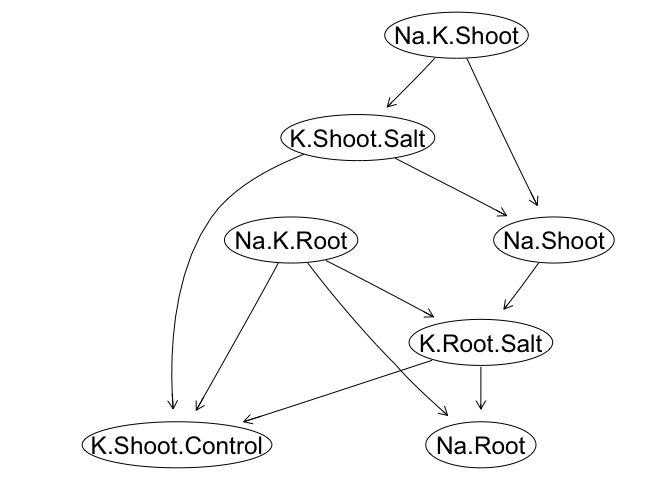### Structure learning: expert prior knowledge + data

Generally, the inference of BN is expected to be purely depended on the data. Under some contexts, prior knowledge about the network structure is available, we could incorporate this prior knowledge into the process of structure learning. We can add and eliminate the edge using arguments of whitelist and blacklist, accordingly.

# Score-based algorithm: Tabu Search
tabu_simple <- tabu(BV)
graphviz.plot(tabu_simple, main = "Tabu", shape = "ellipse", layout = "dot")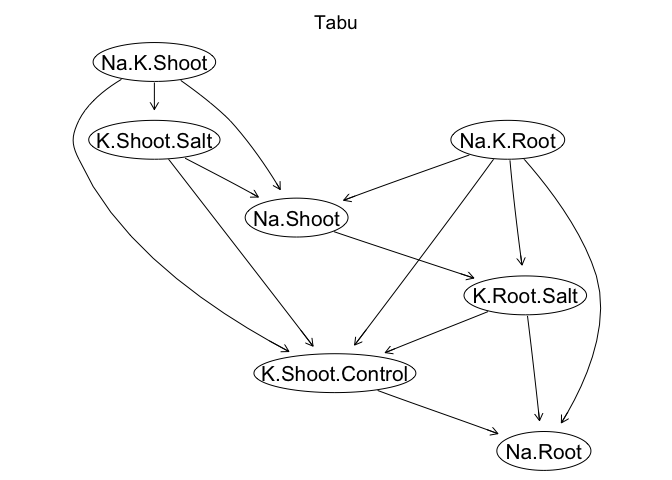# Incorporate subjective prior
whtlist <- c(from = 'Na.K.Root', to = 'Na.K.Shoot')
blklist <- data.frame(from = c("K.Shoot.Salt", "K.Shoot.Control" ),
to = c("K.Shoot.Control", "K.Shoot.Salt"))
# Data + subjective prior
tabu_blk <- tabu(BV, whitelist = whtlist, blacklist = blklist)
graphviz.plot(tabu_blk, main = "Tabu_data-and-prior", shape = "ellipse",
layout = "dot")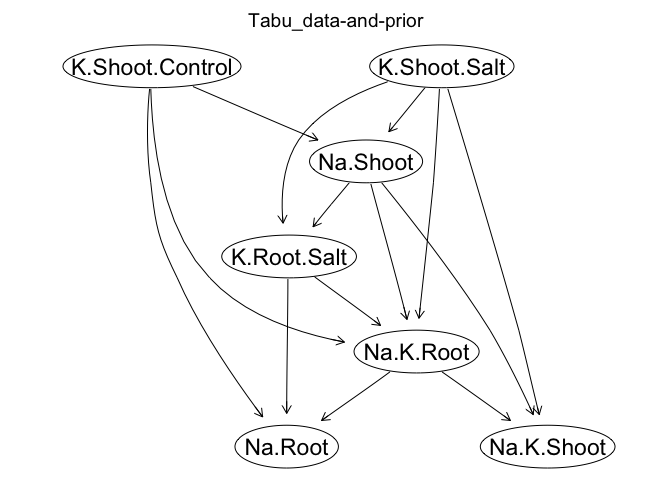## Remove the dependence in EBV with Cholesky Decomposition

Theoretically, BN learning algorithms assume sample independence. The genomic relationship matrix introduced dependencies into the EBV in the multivariate analysis, which potentially serves as a confounder. A transformation of EBV could be done to get adjusted EBV by eliminating the dependencies. We can decompose $$\mathbf{G}$$ into its Cholesky factors $$\mathbf{G} = \mathbf{L} \mathbf{L'}$$. Here, $$\mathbf{L}$$ is an $$n \times n$$ lower triangular matrix. For a single trait we could remove the dependancy from the breeding values $$\mathbf{u}$$ and yield the adjusted breeding values $$\mathbf{u^*}$$ by $$\mathbf{u^*} = \mathbf{L^{-1}} \mathbf{u}$$. When we have multiple traits (t), the dimension of $$\mathbf{u}$$ becomes $$(n \times t) \times 1$$ and $$\mathbf{u^*} = \mathbf{M^{-1}} \mathbf{u}$$, where $$\mathbf{M^{-1}} = \mathbf{I_{(n \times t) \times (n \times t)}} \otimes \mathbf{L^{-1}}$$.

Linv <- solve(t(chol(grm)))
Minv <- kronecker(diag(7), Linv)
BV_adj <- matrix(Minv %*% c(as.matrix(BV)), nrow = 363, ncol = 7)
BV_adj <- as.data.frame(BV_adj)

## Structure learning using adjusted EBV

### Structure learning: data-driven approach

# Score-based algorithm: Tabu Search
graphviz.plot(tabu_simple, main = "Tabu", shape = "ellipse", layout = "dot")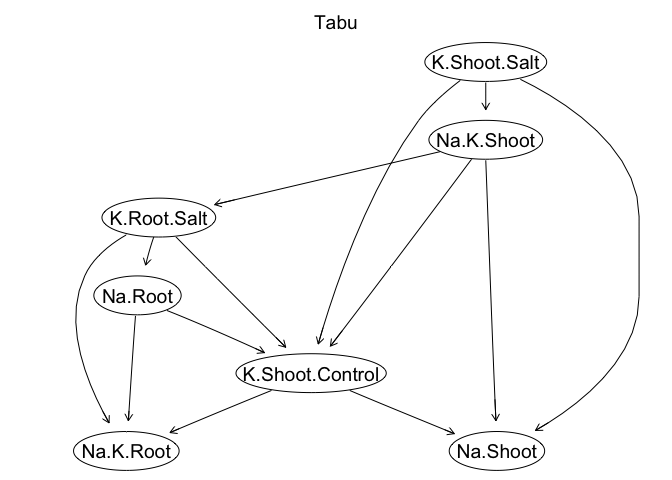# Model Averaging with 500 bootstrap samples to account for uncertainty
set.seed(007)
boot_tabu <- boot.strength (BV_adj, algorithm = "tabu", R = 500)
# Check strength (>= 0.8) and direction (> 0.5)
check_boot(boot_tabu, 0.8, 0.5)
##            from              to strength direction
## 1    Na.K.Shoot        Na.Shoot    1.000 0.8300000
## 2    Na.K.Shoot    K.Shoot.Salt    1.000 0.6400000
## 6    Na.K.Shoot     K.Root.Salt    0.950 0.5105263
## 14 K.Shoot.Salt        Na.Shoot    0.954 0.7442348
## 15 K.Shoot.Salt K.Shoot.Control    1.000 0.9790000
## 35      Na.Root       Na.K.Root    1.000 0.6970000
## 40  K.Root.Salt K.Shoot.Control    0.956 0.9895397
## 41  K.Root.Salt       Na.K.Root    1.000 0.7730000
## 42  K.Root.Salt         Na.Root    1.000 0.6320000
# Average bootstrap samples use threshold = 0.8
ave_model_tabu <- averaged.network(boot_tabu, threshold = 0.8)
graphviz.plot(ave_model_tabu, shape = 'ellipse')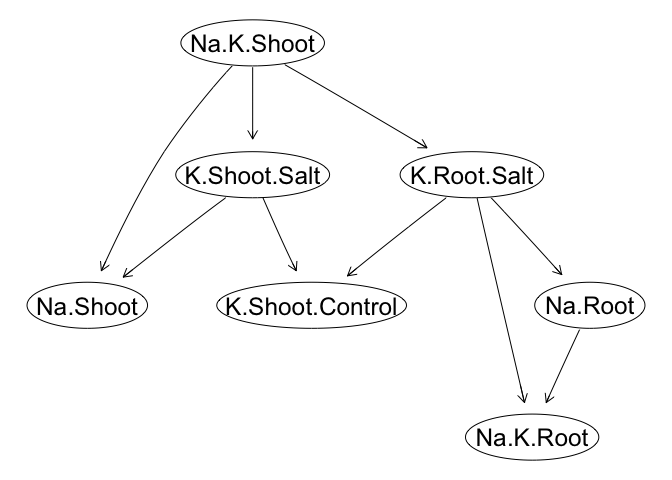### Structure learning: expert prior knowledge + data

# Score-based algorithm: Tabu Search
graphviz.plot(tabu_simple, main = "Tabu", shape = "ellipse", layout = "dot")# Incorporate subjective prior
whtlist <- c(from = 'Na.K.Root', to = 'Na.K.Shoot')
blklist <- data.frame(from = c("K.Shoot.Salt", "K.Shoot.Control" ),
to = c("K.Shoot.Control", "K.Shoot.Salt"))
# Data + subjective prior
tabu_blk <- tabu(BV_adj, whitelist = whtlist, blacklist = blklist)
graphviz.plot(tabu_blk, main = "Tabu_data-and-prior", shape = "ellipse",
layout = "dot")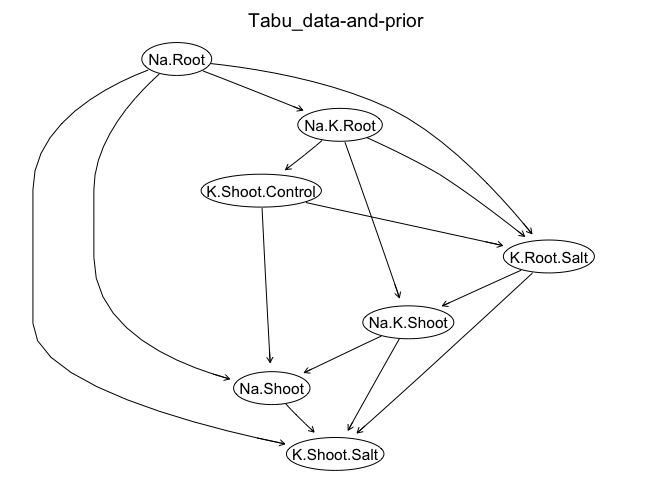## Available BN learning algorithms in bnlearn package

In the above illustrative examples, I used the score-based algorithm of Tabu Search for structure learning. Alternatively, you can use other algorithms by replacing tabu in tabu() and algorithm = "tabu" with the following algorithms. The details can be found in the help page of bnlearn.

• Score-based structure learning algorithms:
• hc: Hill Climbing (HC)
• tabu: Tabu Search (Tabu)
• Constraint-based structure learning algorithms:
• pc.stable : the first practical application of the IC algorithm (the stable version)
• gs: Grow-Shrink (GS)
• iamb: Incremental Association Markov Blanket (IAMB)
• fast.iamb: Fast Incremental Association (Fast-IAMB)
• inter.iamb: Interleaved Incremental Association (Inter-IAMB)
• mmpc: Max-Min Parents & Children (MMPC)
• si.hiton.pc: Semi-Interleaved Hiton-PC (SI-HITON-PC)
• Hybrid structure learning algorithms:
• mmhc: Max-Min Hill Climbing (MMHC)
• rsmax2: General 2-Phase Restricted Maximization (RSMAX2)